Anúncio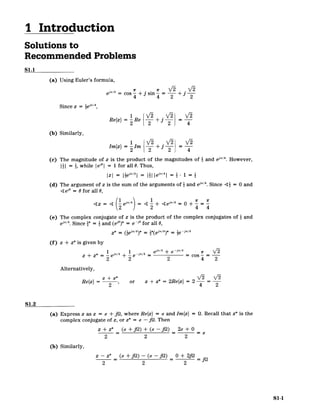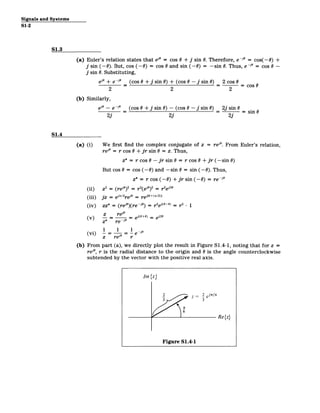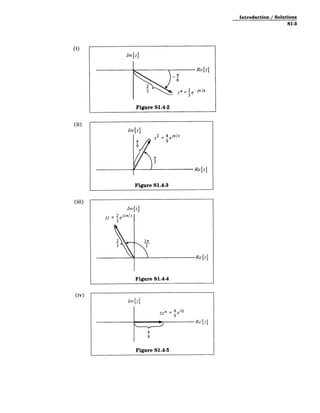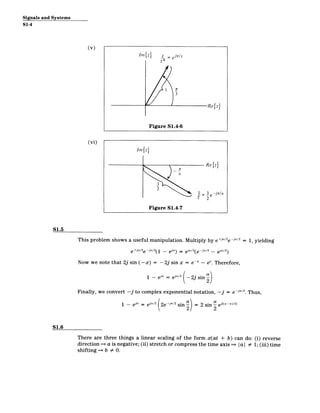Anúncio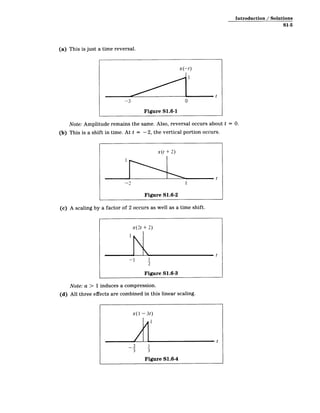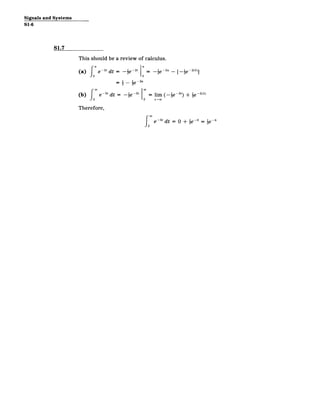Próximos SlideShares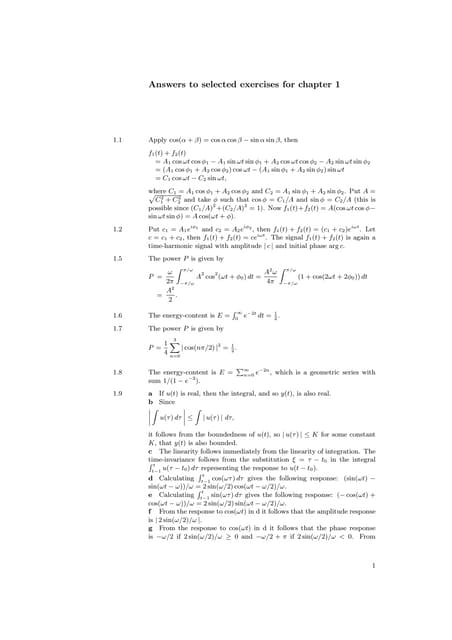Antwoorden fourier and laplace transforms, manual solutions
Carregando em ... 3
1 de 7
Anúncio

### solutions to recommended problems

1. I Introduction Solutions to Recommended Problems S1.1 (a) Using Euler's formula, ,r4 1r IE S r F e cos + j sin-4 2 Since z = iej"/4 Re{z}= Re '2 + 2 (b) Similarly, Im{z}= Im 2 2 4 (c) The magnitude of z is the product of the magnitudes of 2 and eJT/4. However, I| = i, while Iei"| = 1 for all 0. Thus, IzI = e - =I ie 4 | - 1 = (d) The argument of z is the sum of the arguments of i and e'"/4 . Since 4 = 0 and 4 ei" = 0for all 0, = 4 e = + +es"= (e) The complex conjugate of z is the product of the complex conjugates of 1 and ei"/4 . Since * = and (ej")* = e ~i' for all 0, z* =* * 4 =i -i'/4 (f) z + z*is given by 1r/4 1 . 4 ej"/4 + e /4 Vr2/ z + z* =e + e-/4 = = cos ­ 2 2 2 4 2 Alternatively, z+ z* Refz} 2, or z + z* = 2Re{z}= 2 4 2 S1.2 (a) Express z as z = a + jQ, where Re{z} = a and Im{z} = Q. Recall that z* is the complex conjugate of z, or z* = a - jQ. Then z + z* (a+jQ)+ (o -jQ) 2a+ 0 2 2 2 (b) Similarly, z - z* (a +fj) - (a -jQ) _ + 2jQ _. 2 2 2 S1-1
2. Signals and Systems S1-2 S1.3 (a) Euler's relation states that e'" = cos 0 + j sin 0. Therefore, e-o = cos(-0) + j sin (-0). But, cos (-0) = cos 0 and sin (-0) = -sin 0. Thus, e-j" = cos 0 ­ j sin 0. Substituting, e" + e - _ (cos 0 + j sin0) + (cos 0 - j sin0) 2 cos 0 2 2 2 (b) Similarly, ej" - e -_ (cos 0 + j sin0) - (cos 0 - j sin0) 2j sin0 s = =-sin 6 2j 2j 2j S1.4 (a) (i) We first find the complex conjugate of z = re'". From Euler's relation, rej" = r cos 0 + Jr sin 0 = z. Thus, z* = r cos 0 - jr sin 0 = r cos 0 + jr (-sin 0) But cos 0 = cos (-0) and -sin 0 = sin (-0). Thus, z* = r cos (-0) + jr sin (-0) = re -j" (ii) z 2 = (re'") 2 = r2 (ejo) 2 = r2 ei20 (iii) jz = er/2 reo = reO+<,/2) (iv) zz* = (re")(re-jo) = r 2 em-0 )> = r2 1 0 z rej" e o+ )e20 () z* re ~j" = j__= e - e (vi) 0 z re r (b) From part (a), we directly plot the result in Figure S1.4-1, noting that for z = re", r is the radial distance to the origin and 0 is the angle counterclockwise subtended by the vector with the positive real axis. Im{z} 3 jr/6 3 Re(z} Figure S1.4-1
3. Introduction / Solutions S1-3 (i) (ii) Im z) 4 z2 = 4 ej/3 9 9 Rez) Figure S1.4-3 (iii) Im Iz jz= 2 ej27/3 3 2 21r 33 Refz) Figure S1.4-4 (iv) Indz) 4 9 Figure S1.4-5
4. Signals and Systems S1-4 (v) z* Itnzl l= 3 z Rejzj Figure S1.4-6 (vi) ReWjz 7T --6 3 2 1 3 -jn/6 z 2 Figure S1.4-7 S1.5 This problem shows a useful manipulation. Multiply by e+a/ 2 e-ja/2 = 1, yielding 2 e+ja/2 e -ja/2( 1 - eja) ja/ 2 (e -ja/ _ eia/2 ) Now we note that 2j sin (-x) = - 2j sin x = e-x - ex. Therefore, 1 - el" = ei" 2 (-2j sin 2) Finally, we convert -j to complex exponential notation, -j = e -jr/2. Thus, 1 - eia = ea/2 2e -j/2 sin = 2 sin ejt(a-7r)/21 S1.6 There are three things a linear scaling of the form x(at + b) can do: (i) reverse direction => a is negative; (ii) stretch or compress the time axis = a I # 1; (iii) time shifting => b # 0.
5. Introduction / Solutions S1-5 (a) This is just a time reversal. Note: Amplitude remains the same. Also, reversal occurs about t = 0. (b) This is a shift in time. At t = -2, the vertical portion occurs. x(t + 2) t -2 Figure S1.6-2 (c) A scaling by a factor of 2 occurs as well as a time shift. x(2t + 2) t -1 1 2 Figure S1.6-3 Note: a > 1 induces a compression. (d) All three effects are combined in this linear scaling. x(1 -3t) 2 3 1 3 t Figure S1.6-4
6. Signals and Systems S1-6 S1.7 This should be a review of calculus. (a) e 2 tdt = -e - 2 -2a [-e 2(0) = 1 -2a (b) Jf2 e -3' dt = -i 12 = lim (-e t-o0 3 3 1 ) ie-3(2 > Therefore, fe - 3 dt = 0 + 1e 6 56
7. MIT OpenCourseWare http://ocw.mit.edu Resource: Signals and Systems Professor Alan V. Oppenheim The following may not correspond to a particular course on MIT OpenCourseWare, but has been provided by the author as an individual learning resource. For information about citing these materials or our Terms of Use, visit: http://ocw.mit.edu/terms.
Anúncio